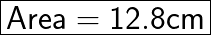WILL GIVE BRAINLIEST!! If the length of a rectangle is decreased by 4 cm and the width is increased by 5 cm, the result will be a squa

Question

WILL GIVE BRAINLIEST!!
If the length of a rectangle is decreased by 4 cm and the width is increased by 5 cm, the result will be a square, the area of which will be 40 cm2 greater than the area of the rectangle. Find the area of the rectangle.

in progress 0
5 months 2021-08-25T17:30:09+00:00 2 Answers 3 views 0

The rectangle is 15 cm by 24 cm, and its area is 360 cm^2.

hope this helps 🙂 plz brainliest

Step-by-step explanation:Step-by-step explanation:

Let,

The length of rectangle = x

The width of rectangle = y

The length of square = x – 4

The width of square = y + 5

Area of rectangle = xy

Area of square = xy + 40

Condition No. 1:

Area of Square = Length of square * Width of square

xy + 40 = (x – 4)(y – 5)

xy + 40 = xy – 5x – 4y + 20

– 5x – 4y = 40 – 20

– 5x – 4y = 20

5x + 4y = -20 ——————(1)

Condition No. 2:

We know that:

Length of square = Width of square

x – 4 = y + 5

x = y + 5 + 4

x = y + 9 —————————(2)

Solution:

Put Eq. (2) in (1)

5 (y + 9) + 4y = -20

5y + 45 + 4y = -20

9y + 45 = -20

9y = -20-45

y = -65 / 9

Now, Put the value of y in Eq. (2)

x = (-65 / 9) + 9

x = (-65 + 81) / 9

x = 16 / 9 cm

Now,

Area of rectangle = xy

Area = ( -65 / 9 ) * ( 16 / 9 )

Area = (-65*16) / (9*9)

Area = -1040/81

Area = 12.8 cm² (Neglecting -ve sign)Hope this helped!